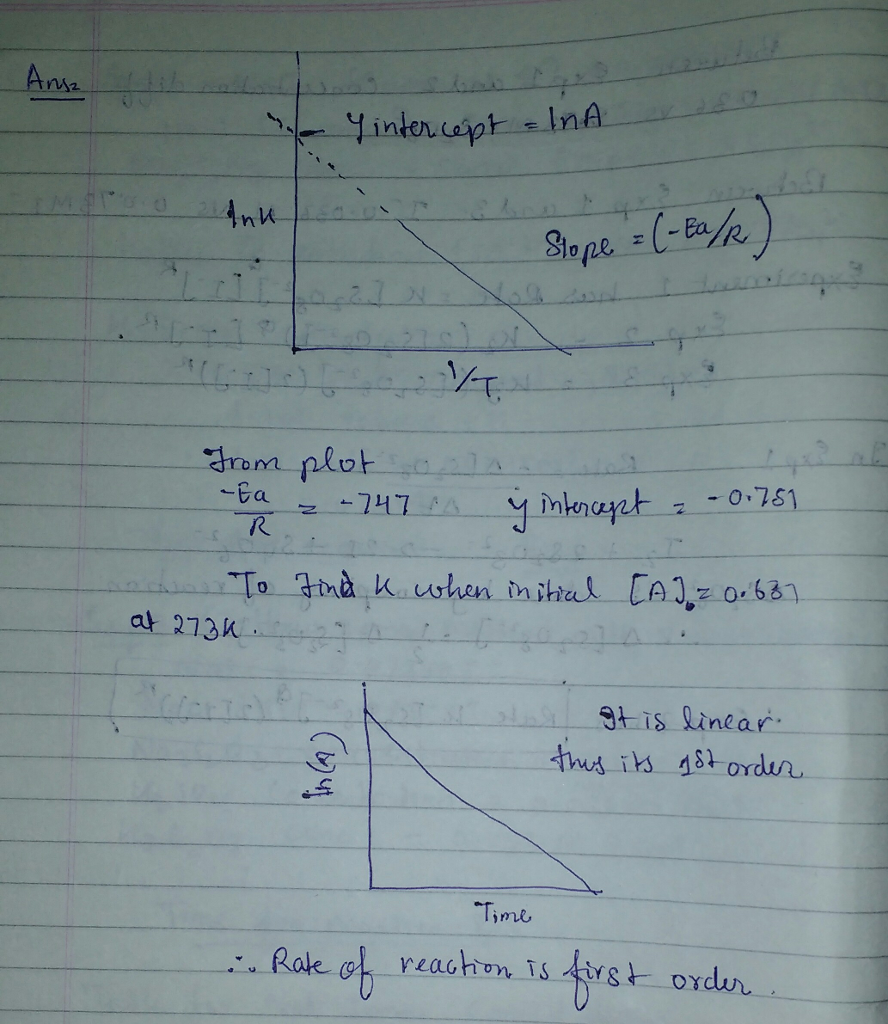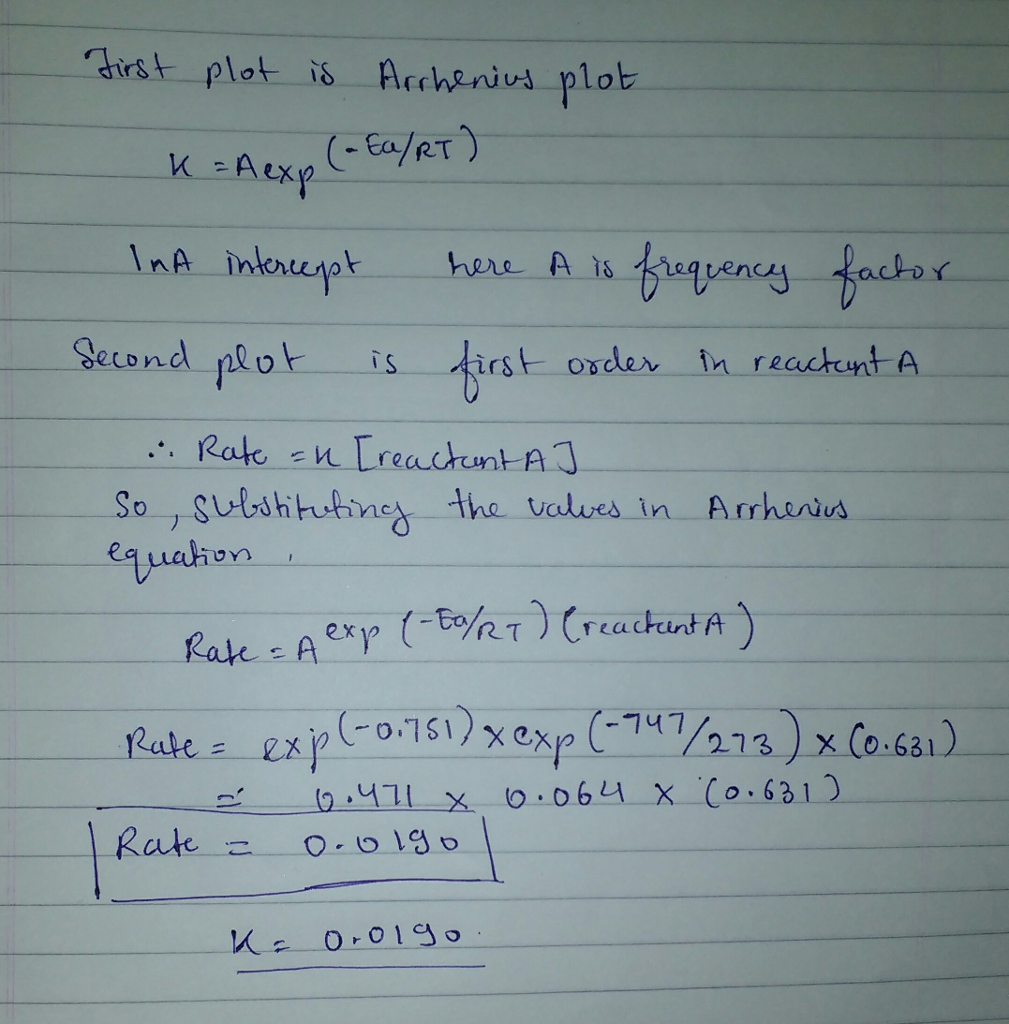# Question & Answer: The reaction A → products was studied at a series of different temperatures. A plot of ln(k) vs……

The reaction A → products was studied at a series of different temperatures. A plot of ln(k) vs. 1/T gave a straight line relationship with a slope of -747 and a y-intercept of -0.751. Additionally, a study of the concentration of A with respect to time showed that only a plot of ln[A] vs. time gave a straight line relationship. What is the initial rate of this reaction when [A] = 0.631 at 273 K?